Next: Primitive roots Up: Introduction to Number Theory Previous: The Greatest Common Divisor   Contents

## Powers modulo a prime

The sequence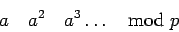has many applications in cryptography. Before looking at theoretical properties, the example below (done using a pocket calculator) should make clear that it is practical to compute these numbers, even when many digits are involved.

Suppose we want to compute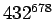mod 987. The basic trick is to start with a number and keep squaring: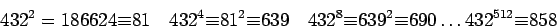Since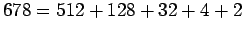,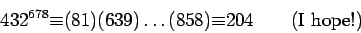Calculations with exponents involve not-too-many multiplications. If the numbers have several hundred digits, however, it is necessary to design special subroutines to do the multiplications (see Knuth, volume 2).

Let us look at the sequence of powers of 2 mod 11:Each number from 1 to 10 appears in the sequence.

Theorem 5   Let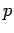be a prime. There is an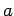such that for every, there is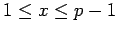such that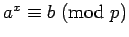.

It is not always the case that. The powers of 2 mod 7 are 2, 4, 1 after which the sequence repeats and we never get 3, 5, or 6.

The proof of Theorem 5 is long, and we will give it in section 2.5. For now, we want to look at some of its consequences.

Corollary 6   Letbe as in Theorem 5. Then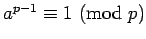.

Proof: We know thatfor some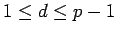. If, the sequence of powers ofwould start repeating before we got all the numbers: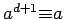,, etc. height 8pt width 4pt

Corollary 7   For any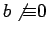,.

Proof: Letbe as in Theorem 5. Using Corollary 6Corollary 8   If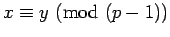, then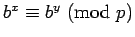Proof: For some integer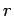,and by Corollary 7Lemma 9   Let,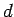the smallest positive number such that. Then for anywith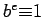divides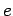evenly. In particular, by Corollary 7,dividesevenly.

Proof: Ifdoes not divide, thenfor some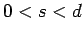, but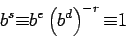would contradict the definition of. height 8pt width 4ptNext: Primitive roots Up: Introduction to Number Theory Previous: The Greatest Common Divisor   Contents
Translated from LaTeX by Scott Sutherland
2002-12-14